### Home > PC > Chapter 2 > Lesson 2.3.6 > Problem2-143

2-143.
1. Suppose we want to find the area under the function f(x) = 2x on the interval 1 ≤ x ≤ 3. Homework Help ✎

1. Sketch a graph of the curve shading the area of the region you are to find.

2. If left-endpoint rectangles are used will the estimate be too low or too high. Justify your answer.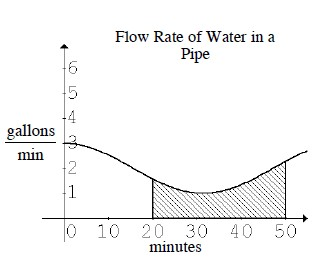3. If you used right-endpoint rectangles to estimate the area, will that estimate be too low or too high. Justify your answer.

4. Use your program to find an estimate using 20 left- and right-endpoint rectangles.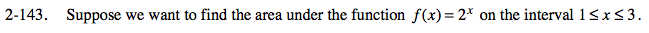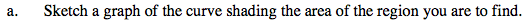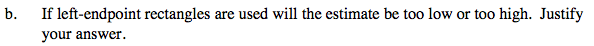Too low, as rectangles will be under the curve.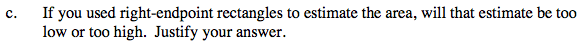Too high, as rectangles will be above the curve.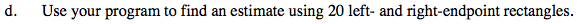Left = 8.360, Right = 8.960